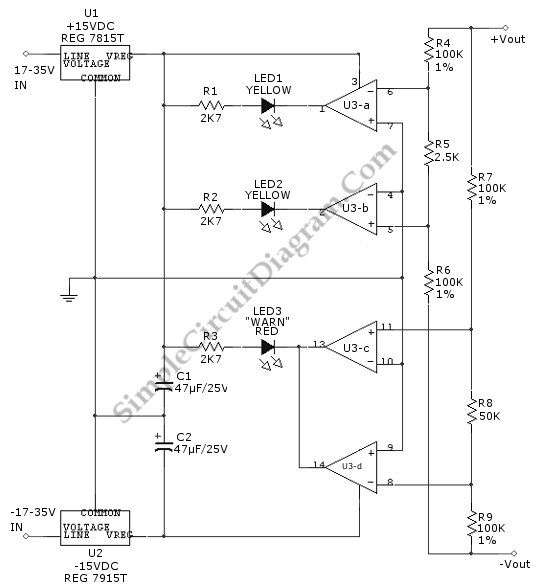# Balance Indicator for Symmetric Power Supply

Balance indicator for power supply show if a symmetric  power supply is really symmetric or not. With two comparator pairs from a LM339N quad comparator we can make a such indicator. One pair drives the red warn LED3 and the other pair drive the yellow negative(-) and positive (+). The power supply of this circuit is an unregulated voltage. The two parallel resistor-divider strings give switching inputs the for the four comparators. Both strings have their ends tied between the negative output terminals and the power positive terminals. Here is the circuit:R5, R6 and R4 are first string which will divide the input voltage in half, with output taps at 0.5%. R8, R9, and R7 also divide the input voltage in half but taps at +10%. The two comparator are driven by the 0.5% R6/R4/R5 string to control the negative and positive indicators(LED2 and LED1). The LED2 will not light if the positive supply is lower than the negative. The positive indicator will not go off if the negative the positive supply still 0.5% higher than negative supply. Both indicators will on together when the two supply are in 1% or better balance.

The warn indicator is controlled by two comparators those are driven by + 10% R8/R7/R9 string. When the either supply reach 10% or more higher than the other, the red LED3 will on caused by one of two comparator that switch its output low. This circuit can be added by wired OR connections because the LM339 has opened-collector outputs. There is a band where neither comparators output is low and LED remains off.